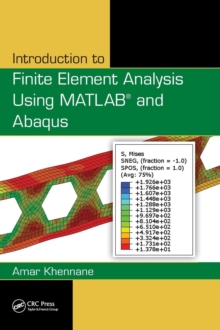# Introduction to Finite Element Analysis Using MATLAB (R) and Abaqus Hardback

#### Description

There are some books that target the theory of the finite element, while others focus on the programming side of things.

Introduction to Finite Element Analysis Using MATLAB (R) and Abaqus accomplishes both.

This book teaches the first principles of the finite element method.

It presents the theory of the finite element method while maintaining a balance between its mathematical formulation, programming implementation, and application using commercial software.

The computer implementation is carried out using MATLAB, while the practical applications are carried out in both MATLAB and Abaqus.

MATLAB is a high-level language specially designed for dealing with matrices, making it particularly suited for programming the finite element method, while Abaqus is a suite of commercial finite element software. Includes more than 100 tables, photographs, and figuresProvides MATLAB codes to generate contour plots for sample resultsIntroduction to Finite Element Analysis Using MATLAB and Abaqus introduces and explains theory in each chapter, and provides corresponding examples.

It offers introductory notes and provides matrix structural analysis for trusses, beams, and frames.

The book examines the theories of stress and strain and the relationships between them.

The author then covers weighted residual methods and finite element approximation and numerical integration.

He presents the finite element formulation for plane stress/strain problems, introduces axisymmetric problems, and highlights the theory of plates.

The text supplies step-by-step procedures for solving problems with Abaqus interactive and keyword editions.

The described procedures are implemented as MATLAB codes and Abaqus files can be found on the CRC Press website.

#### Information

• Format: Hardback
• Pages: 487 pages, Approx. 690 to 725 equations; 6 Tables, black and white; 360 Illustrations, black and whi
• Publisher: Taylor & Francis Inc
• Publication Date:
• Category: Numerical analysis
• ISBN: 9781466580206

£125.00

£115.19

on all orders

###### Pick up orders

from local bookshops